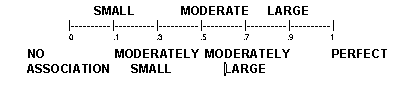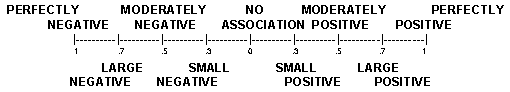SPSS

SPSS is a pc based database which computes most of the statistics covered in this class. Other outputs are available, but will not be covered. To learn more, there are resource guides for SPSS.

After logging on, run SPSS. Then access the SPSS database containing the information represented by the Hypothesis being studied. Choose some variables to examine. Use the Explore feature under Statistics to see a histogram of the data. Choose frequencies or descriptives for more detail. SPSS will temporarily save all outputs to a temporary file named SPSS.LIS. After each computation , data will be presented in an OUTPUT box, when this box is highlighted (the top bar is blue) save the results by inserting a disc into the A: drive and under the File header choose save as. Just choose a name, the program will automatically give it the proper extension. After editing (by simply typing, cutting, or deleting in the output box) all extraneous results, print the file by choosing Print under the File header.

FREQUENCY VARIATION TABLE

Command: FREQUENCIES VAR = SIGIVE/STATISTICS MEAN MEDIAN MODE.

SPSS/PC+

SIGIVE DATE DELIBERATELY BECAME INTOXICATED IN ORDER TO HAVE SEX

 Value Label Value Frequency Percent Valid Percent Cum Percent yes 1 45 24.7 25.00 25.00 no 2 135 74.2 75.00 100.00 9 2 1.1 MISSING TOTAL 182 100.0 100.0

Mean 1.750 Median 2.000 Mode 2.000

Valid Cases 180 Missing Cases 2

*Data collected at SDSU during the Spring semester, 1992.

There are six columns of numbers in the frequency table. The first is the Value Label column. This includes the title of each variable category. To its right is the Value column. The value is the numerical code given to each category. If the category is interval, it is usually the same as the value label (the value label may not be included in these cases). However, if the categories are ordinal or nominal, the data must be coded and inputted numerically in order to use SPSS.

Once the categories are identified, a third column labeled Frequency is visible. This is the number of cases that are in each category. In the shaded example, 45 cases are in the "yes" category. Continuing to the right, find the Percent column. This is the frequency divided by the total sample number. This column includes all cases, even those that did not respond to this item on their surveys (Missing cases). The subsequent Valid Percent column deletes the missing cases and produces the percentage of cases that responded to that particular survey question. The example shows that 25% of those responding said "yes"' to the question. And finally, the Cum Percent column. This column adds the percent of the previous categories listed and the current category identified by the value label. In the example, the second category "no" includes the proportion of cases that responded "no" plus the proportion of cases that responded to the previously listed category "yes". Because the sample has only two categories, this cumulative percentage is 100%.

In the Summarize frequencies, STATISTICS MEAN MEDIAN MODE are given. The above data is nominal, so the MODE is the only valid descriptive. If the box STATISTICS ALL had been checked, SPSS would have provided a readout that included all the univariate statistics SPSS offers. Variation Ratio and Leik's Measure are not available, but Standard Deviation, measures for Kurtosis, Skewness and Variance are. Remember that not all the data will be valid based upon the assumptions of the statistics and the level of measurement of the variable.

Because all the data is coded numerically, the computer cannot determine the level of measurement. The researcher must determine the level of measurement and appropriate statistic to use!

CROSSTABULATION TABLE

Command: CROSSTABS TABLES=SIGIVE BY CAT/OPTIONS=3,4,5/STATISTICS=ALL.

SPSS/PC+

Crosstabulation: SIGIVE DATE DELIBERATELY BECAME INTOXICATED IN ORDER TO HAVE SEX

by CAT FRATERNITY MEMBERSHIP

 CAT Count Row Pct Col Pct Tot Pct FRAT MEMBER 1 NON-GREEK 4 Row Total SIGIVE YES 1 14 31.8 36.8 7.9 30 68.2 21.6 16.9 44 24.9 ROWS NO 2 24 18.0 63.2 13.6 109 82.0 78.4 61.6 133 75.1 COLUMNS Column Total 38 21.5 139 78.5 177 100.0

Chi-Square D.F. Significance Min E.F. Cells with E.F.<5

2.94791 1 .0860 9.446 None

3.71998 1 .0538 (Before Yates Correction)

With SIGIVE With CAT

Statistic Symmetric Dependent Dependent

Lambda .00000 .00000 .00000

Uncertainty Coefficient .01830 .01764 .01902

Somers' D .14478 .15259 .13773

Eta .14497 .14497

Statistic Value Significance

Phi .14497

Contingency Coefficient .14347

Kendall's Tau B .10291 .0272

Kendall's Tau C .16385 .0272

Pearson's R .14497 .0271

Gamma .35886

Number of Missing Observations = 5

THE CELLS

There are four cells in the above SPSS crosstabulation output that designate the four possible categories each survey respondent could fall into. The shaded upper left cell are those respondents who have had a date that became deliberately intoxicated in order to have sex and are part of a fraternity. Each of these cells has four entries: The first is the count number (Count). This is the absolute number of cases or respondents with both the row and column characteristics. In the shaded example, 14 cases have stated "yes" they have had dates that became deliberately intoxicated in order to have sex and are fraternity members. Immediately below the count number is the row percentage (Row Pct). The row percentage identifies that the 14 people represent 31.8% of those that have dates who deliberately became intoxicated are also fraternity members. Next is the column percentage (Col Pct) which identifies that 36.8% of the fraternity members have had dates who deliberately became intoxicated. The final number is the total percentage (Tot Pct) which identifies that of all the respondents in the table, 7.9% have had dates who deliberately became intoxicated and belong to a fraternity.

THE STATISTICS

The shaded statistics are those covered in this semester. Remember, since all data is coded in numbers, the researcher must determine the appropriate statistic to use. Identify whether or not the data meets the assumptions for that statistic, the Explore feature under Statistics is very helpful for this.

The example has variables which are nominal, dichotomous and discrete. This means use Phi Coefficient to identify the relationship. Phi Coefficient equals .14497. This means there is a small positive relationship between being a fraternity member and having had a date who deliberately became intoxicated in order to have sex.

Next, determine whether or not one can generalize this relationship to the population. Nominal data uses Chi Square to determine its probability values. The significance is .0860 (with Yates Correction). Therefore one cannot generalize the findings. Note that the Chi Square statistic has a column labeled "cells with E.F.< 5." This column should ideally be NONE, but if it is under 20%, Chi Square will still be valid.

MEASURES OF CORRELATION

LAMBDA: Used for measuring relationships between nominal variables. s has no hypothetical direction. a assumes a hypothetical direction. SPSS figures asymmetrical a for both variables. Interpretations for s: "There is a ______ association between (variable 1) and (variable 2)" or convert to a percent and include in the statement, "There is a ____% improvement when trying to predict both variables simultaneously from the knowledge of each other".

Interpretations for A: "There is a_______association between (variable 1) and (variable 2)." or convert to a percent and include in the statement, "There is a ____% improvement when trying to predict (dependent variable) from the knowledge of (independent variable)."ETA: Used for measuring relationships between nominal and interval variables. Interpretation for : "There is ______association between (variable 1) and (variable 2)."Interpretation for 2: Convert to a percent and include in the statement, "_____% of the variance in (interval variable) can be explained by (nominal variable)." or 1 - 2: Convert to a percent and include in the statement, "_____% of the variance in (interval variable) cannot be explained by (nominal variable)."

PHI COEFFICIENT: Used for measuring relationships between nominal variables that are both discrete and dichotomous. Interpretation for : "There is a ______ association between (variable 1 category in upper left corner) and (variable 2 category in upper left corner)."a b *Remember! for the categories of cell "a" will be identical for the categories fo cell "d" and exactly opposite c d for the categories of cells "b" & "c".

TAU B: Used for measuring relationships between ordinal variables that have an equal number of categories. The crosstabulation table will be square shaped. Interpretation for b: "There is a ______ association between (variable 1) and (variable 2)."

TAU C: Used for measuring relationships between ordinal variables that do not have an equal number of categories. The crosstabulation table will be rectangular. This class did not cover c, interpret it the same as b.

PEARSON'S r : Used for measuring relationships between interval variables. Assumes a linear relationship and homoscedasticity. Interpretation for r: "There is a ______ association between (variable 1) and (variable 2)."For r2: Convert to a percent and include in the statement "_____% of the variance in (variable 1) can be explained by (variable 2)." or vice versa. For 1 - r2: Convert to a percent and include in the statement, "_____% of the variance in (variable 1) cannot be explained by (variable 2)." or vice versa.

GAMMA: Used for measuring relationships between ordinal variables. Interpretation for G: Convert to a percent and include in the statements, "There is a ________ association between (variable 1) and (variable 2)." or for a positive relationship - "There is ____% more agreement than disagreement in the rank order of (variable 1) and (variable 2)" and for a negative relationship - "There is _____% more disagreement than agreement in the rank order of (variable 1) and (variable 2)."MEASURES OF INFERENTIAL PROBABILITY

CHI SQUARE SIGNIFICANCE: Used for measuring probability values of nominal data. Assumes independent variables and fe 5. Probability values equal to or less than .05 can be generalized to the population.

SIGNIFICANCE OF TAU: Used for measuring probability values of ordinal data. Assumes a sample total greater than or equal to 10. Probability values equal to or less than .05 can be generalized to the population.

SIGNIFICANCE OF PEARSON'S R: Used for measuring probability values of interval data. Assumes a sample total greater than or equal to 10, a linear relationship, homoscedasticity, and a normal distribution in the whole population. Probability values equal to or less than .05 can be generalized to the population.

SPSS project